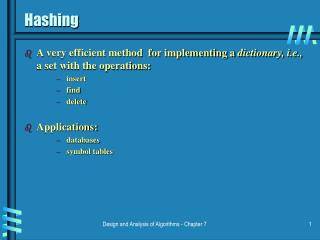# Hashing - PowerPoint PPT PresentationDownload PresentationHashing

Download Presentation## Hashing

- - - - - - - - - - - - - - - - - - - - - - - - - - - E N D - - - - - - - - - - - - - - - - - - - - - - - - - - -
##### Presentation Transcript

1. Hashing • A very efficient method for implementing a dictionary, i.e., a set with the operations: • insert • find • delete • Applications: • databases • symbol tables Design and Analysis of Algorithms - Chapter 7

2. Hash tables and hash functions • Hash table: an array with indices that correspond to buckets • Hash function: determines the bucket for each record • Example: student records, key=SSN. Hash function: h(k) = k mod m (k is a key and m is the number of buckets) • if m = 1000, where is record with SSN= 315-17-4251 stored? • Hash function must: • be easy to compute • distribute keys evenly throughout the table Design and Analysis of Algorithms - Chapter 7

3. Collisions • If h(k1) = h(k2) then there is a collision. • Good hash functions result in fewer collisions. • Collisions can never be completely eliminated. • Two types handle collisions differently: • Open hashing - bucket points to linked list of all keys hashing to it. • Closed hashing – • one key per bucket • in case of collision, find another bucket for one of the keys (need Collision resolution strategy) • linear probing: use next bucket • double hashing: use second hash function to compute increment Design and Analysis of Algorithms - Chapter 7

4. Open hashing • If hash function distributes keys uniformly, average length of linked list will be n/m • Average number of probes = 1+α/2 • Worst-case is still linear! • Open hashing still works if n>m. Design and Analysis of Algorithms - Chapter 7

5. Closed hashing • Does not work if n>m. • Avoids pointers. • Deletions are not straightforward. • Number of probes to insert/find/delete a key depends on load factor α = n/m (hash table density) • successful search: (½) (1+ 1/(1- α)) • unsuccessful search: (½) (1+ 1/(1- α)²) • As the table gets filled (α approaches 1), number of probes increases dramatically: Design and Analysis of Algorithms - Chapter 7